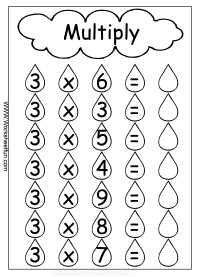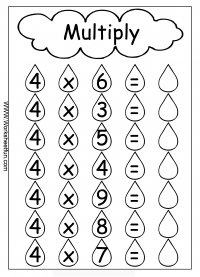Multiplication- Multiplication Activity – Multiply by 2, 3, 4, 5, 6, 7, 8, 9, 10 & 11 – Eleven Worksheets

Cool Multiplication Activity
Multiply by 2, 3, 4, 5, 6, 7, 8, 9, 10 & 11Cool Math Activity

Multiplication Worksheets

Multiplication

Multiplication – Basic Facts

Multiplication – Cubes

Multiplication – Horizontal

Multiplication – Quiz

Multiplication – Test

Multiplication – Vertical

Multiplication Target Circles

Multiplication Word Problems

Multiplication-1 Digit

Multiplication-2 Digit by 2 Digit

Multiplication-3 Digit by 1 Digit

Multiplication-3 Digit by 2 Digit